# Color Codes Conversion

In this tutorial , we will see that how different color codes can be combined to make other colors, and how we can covert RGB color codes to hex and vice versa.

## Different color codes

All the colors here are of the 24 bit format, that means each color has 8 bits of red , 8 bits of green , 8 bits of blue , in it. Or we can say each color has three different portions. You just have to change the quantity of these three portions to make any color.

## Color:Black

### Image: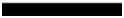(0,0,0)

### Explanation:

As it has been explained in the previous tutorials , that in an 8-bit format , 0 refers to black. So if we have to make a pure black color , we have to make all the three portion of R , G , B to 0.

## Color:White

### Image: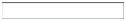(255,255,255)

### Explanation:

Since each portion of R,G,B is an 8 bit portion. So in 8-bit , the white color is formed by 255. It is explained in the tutorial of pixel. So in order to make a white color we set each portion to 255 and thats how we got a white color. By setting each of the value to 255 , we get overall value of 255 , thats make the color white.

## Color:Red

### Image:(255,0,0)

### Explanation:

Since we need only red color , so we zero out the rest of the two portions which are green and blue , and we set the red portion to its maximum which is 255.

## Color:Green

### Image: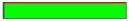(0,255,0)

### Explanation:

Since we need only green color , so we zero out the rest of the two portions which are red and blue , and we set the green portion to its maximum which is 255.

## Color: Blue

### Image: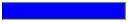(0,0,255)

### Explanation:

Since we need only blue color , so we zero out the rest of the two portions which are red and green , and we set the blue portion to its maximum which is 255

## Gray color:

### Image:(128,128,128)

### Explanation:

As we have already defined in our tutorial of pixel , that gray color Is actually the mid point. In an 8-bit format , the mid point is 128 or 127. In this case we choose 128. So we set each of the portion to its mid point which is 128 , and that results in overall mid value and we got gray color.

## CMYK color model:

CMYK is another color model where c stands for cyan , m stands for magenta , y stands for yellow , and k for black. CMYK model is commonly used in color printers in which there are two carters of color is used. One consist of CMY and other consist of black color.

The colors of CMY can also made from changing the quantity or portion of red , green and blue.

## Color: Cyan

### Image:(0,255,255)

### Explanation:

Cyan color is formed from the combination of two different colors which are Green and blue. So we set those two to maximum and we zero out the portion of red. And we get cyan color.

## Color: Magenta

### Image:(255,0,255)

### Explanation:

Magenta color is formed from the combination of two different colors which are Red and Blue. So we set those two to maximum and we zero out the portion of green. And we get magenta color.

## Color: Yellow

### Image: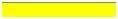(255,255,0)

### Explanation:

Yellow color is formed from the combination of two different colors which are Red and Green. So we set those two to maximum and we zero out the portion of blue. And we get yellow color.

## Conversion

Now we will see that how color are converted are from one format to another.

### Conversion from RGB to Hex code:

Conversion from Hex to rgb is done through this method:

1. Take a color. E.g: White = (255, 255 , 255).

2. Take the first portion e.g 255.

3. Divide it by 16. Like this:

4.5. Take the two numbers below line , the factor , and the remainder. In this case it is 15 � 15 which is FF.

6. Repeat the step 2 for the next two portions.

7. Combine all the hex code into one.

### Conversion from Hex to RGB:

Conversion from hex code to rgb decimal format is done in this way.

Take a hex number. E.g: #FFFFFF

Break this number into 3 parts: FF FF FF

Take the first part and separate its components: F F

Convert each of the part separately into binary: (1111) ( 1111)

Now combine the individual binaries into one: 11111111

Convert this binary into decimal: 255

Now repeat step 2 , two more times.

The value comes in the first step is R , second one is G, and the third one belongs to B.

Answer: ( 255 , 255 , 255 )

## Common colors and their Hex code has been given in this table.

ColorHex Code
Black#000000
White#FFFFFF
Gray#808080
Red#FF0000
Green#00FF00
Blue#0000FF
Cyan#00FFFF
Magenta#FF00FF
Yellow#FFFF00